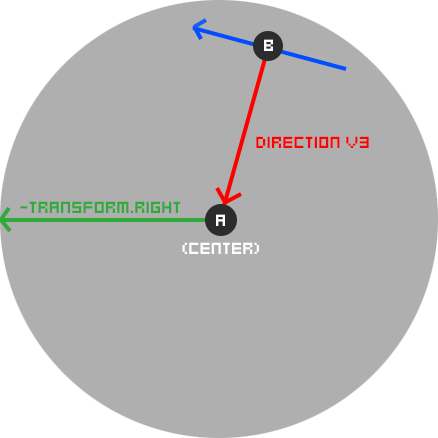# How to calculate vector perpendicular to a direction vector?

Hello,

I am trying to get the direction of the vector perpendicular to the direction vector of 2 points(A,B) illustrated below. I understand a cross product requires a reference vector like transform.right to work, but I get weird behavior when the direction(dir) vector is equal to the reference vector transform.right: the rigidbody2d I am moving with it just wobbles back and forth.

P.S. using the rigidbodies transform.right would be good, but it’s always rotating, so that can’t work.

Any physics-related suggestions are appreciated!

``````	void Rotate(Rigidbody2D rb)
{
Vector3 dir = rb.transform.position - transform.position;
dir.Normalize();
Vector3 side = Vector3.Cross(dir, -transform.right);
Vector3 cross = Vector3.Cross(dir, side);
}
``````If it’s in 2D you can just swap the components and negate one:

``````blueVector.x = redVector.y;
blueVector.y = -redVector.x;
``````

(You’ll probably then want to normalise it so it’s a consistent magnitude)

FWIW, the cross product will give you a vector perpendicular to both the input vectors, so it’s useless in 2D.

[EDIT] BTW, if you keep adding that tangential velocity it will spiral rapidly outwards, even with damping. I’m guessing you might want it to orbit, in which case you’ll need a “gravity” force to stop it flying off.

In 2D:
direction vector = (x,y) =>
normal vector = (-y,x) or (y,-x)

In 3D:
direction vector = (x,y,z) =>
normal vector (in plane y, y stay) = (-z,y,x) or (z,y,-x)